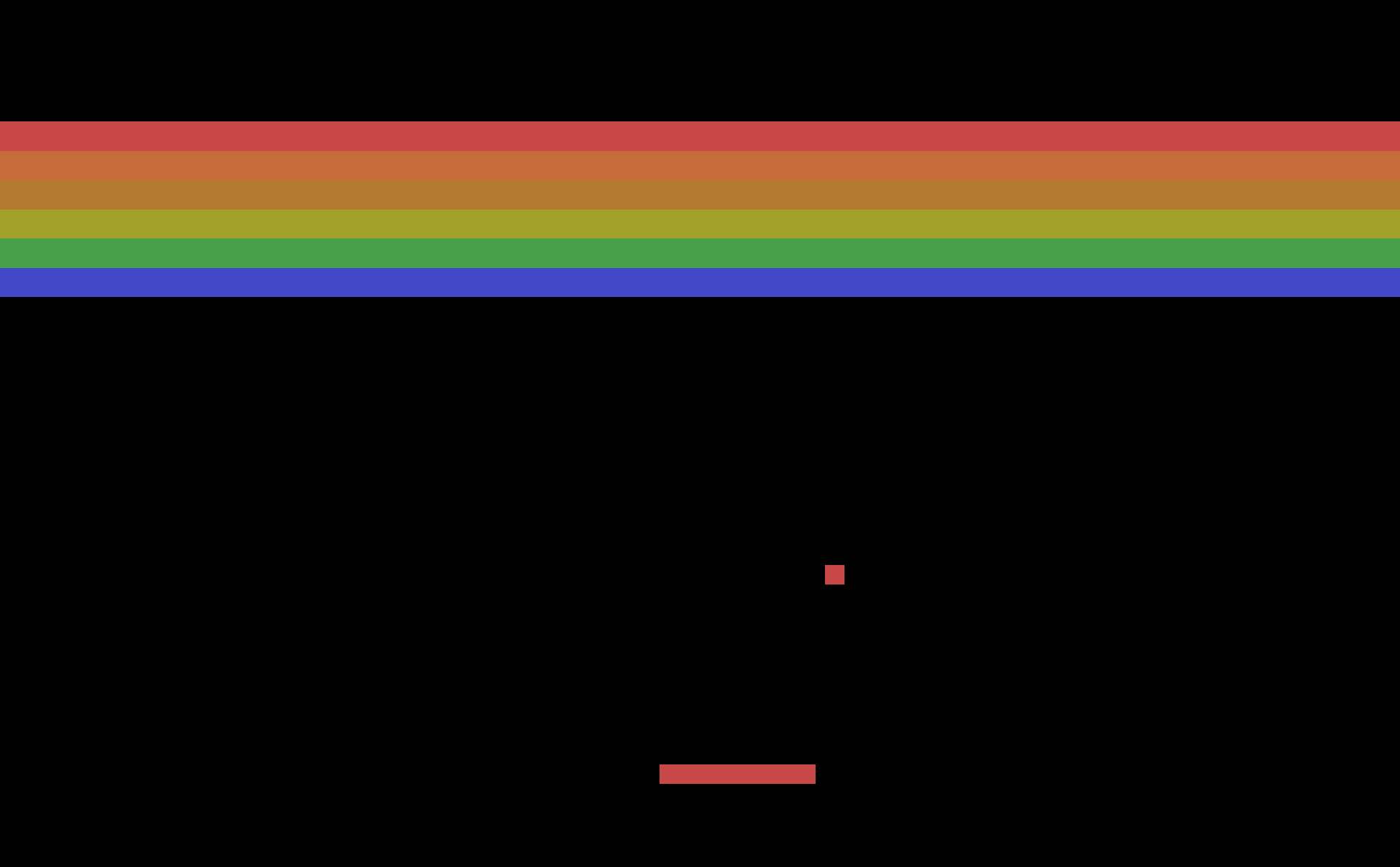In this post, I will demonstrate and explain Reinforcement Learning code I developed. You will learn how one can train an AI agent to master Atari games and understand the technology behind DeepMind’s AlphaGo, the first computer program to defeat professional human Go player. This will be fun and, surprisingly, simple, so let’s dive in.

## OpenAI Gym

OpenAI’s gym is a toolkit for developing and comparing reinforcement learning algorithms. Big standardized datasets have proven pivotal in development of deep learning algorithms. Similarly, collection of test problems, environments, as provided by gym aids in evaluation of reinforcement learning algorithms when an agent learns to take actions in response to observations from environment to “solve it”. All that comes below is enabled by the work of guys at OpenAI. Hat tip to them.

## The CartPole Problem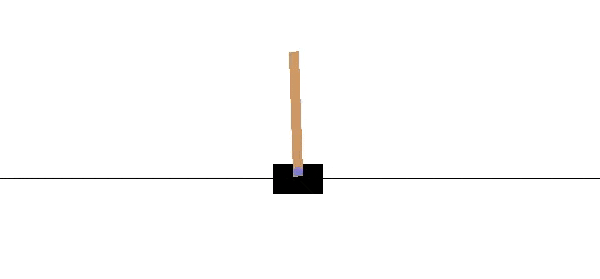Trained agent skillfully balancing the pole on the cart

The CartPole-v0 environment is a reinforcement learning (RL) equivalent of Hello World!. As such, it is sufficiently simple to get us started. It is a form of the classic control problem of inverted pendulum where we balance a pole by moving a cart it is attached to either left or right. The environment is considered solved when the average reward is greater than or equal to 195.0 over 100 consecutive trials. You can check the environment’s spec’s at its wiki.

### SARSA

To train an agent for this environment, I will use the SARSA reinforcement learning algorithm. This is an on-policy learning algorithm (which means that it uses the same policy to generate both current $a_t$ as well as the next action $a_{t+1}$). The name SARSA comes from the fact that the update rule for a Q-value depends on the tuple $(s_t, a_t, r_t, s_{t+1}, a_{t+1})$, where $s_t$, $a_t$ and $r_t$ are state, action and reward at time $t$ respectively.

The concept of Q-value is simple one. You just need to understand that we need a value to optimize that describes the reward the agent has harvested during a period of gameplay. First, define Value function $V^{\pi}(s)$ which is an expected sum of discounted rewards upon starting at state $s$ and selecting actions according to policy $\pi$:

$V^{\pi}(s) = E[R(s_0)+\gamma R(s_1)+\gamma^2 R(s_2) + ... | s_0=s,\pi].$

$R(\cdot)$ gives the immediate value of reward and the discount factor $\gamma$ expresses how much we care about past values of it. Q-value function is then just V function for a particular action $a_t$ for each state $s_t$. They are related with:

$V^\pi(s) = \sum_{a \in A} \pi (a|s) * Q^\pi(a,s).$

Given the above definitions, the SARSA algorithm updates the Q-value by an error-term adjusted by the learning rate $\alpha$ as follows:

$Q(s_t,a_t)\leftarrow Q(s_t,a_t)+\alpha[r_{t+1}+\gamma Q(s_{t+1},a_{t+1})-Q(s_t,a_t)]$

In my implementation I approximate the Q-values for each action with a linear function and select an action with $argmax(w^Ts)$.

Here is the implementation (full code here):

### Results

The algorithm works very well and we are able to solve the environment as early as after 60 or so episodes.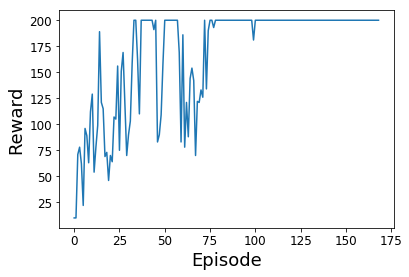Solving the CartPole problem

## Atari Games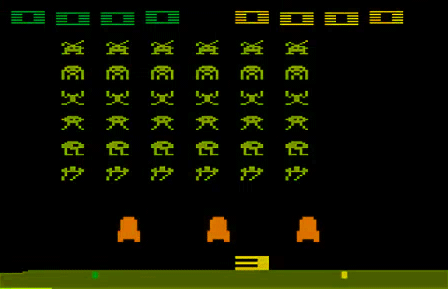Example of an Atari Game (Space Invaders)

After successfully training an agent on a simple problem, I jumped on something more complex. That is, teaching agent how to master Atari games. I focused on the game of Breakout. This problem is very different from the previous one in that as input to our model we receive images of the game screen. Learning something from this complex input requires a model that is capable of abstracting features from it. This is why I decided to harness the power of a deep convolutional neural network.

### The Deep Model

For my implementation, I chose the DQN model and algorithm that was used by DeepMind to learn how to play Go. I extended it by few recent developments from the field of deep reinforcement learning including the dueling DQN and double DQN. I also used the Prioritized Experience Replay Memory to train the agent on samples from past to prevent it forgetting about past experiences. The full code is available at my GitHub.

Here is the model in code:

You will see that the model architecture is pretty big. This is why the researchers at DeepMind had to train it for 50 million frames to achieve great results. You will notice that I am using a custom loss function. It allows me to update Q-values only for actions that were observed and also avoids the problem of weighting outliers too heavily that otherwise occurs with mean squared loss.

The Huber loss is implemented as follows:

### Epsilon-Greedy Policy

I used RMSProp optimizer as it was also the one used in the original paper. Once the memory has been initialized with sufficient number of samples, then the network is retrained on each step by sampling a random batch from memory. The policy implemented is Epsilon Greedy Policy and it differs from the previously described SARSA algorithm in that it is implemented as an off-policy algorithm. That is, it chooses the next action in greedy fashion, in order to maximize the next reward.

### Checkpointing and Visualizations

Initially, I was getting hardly any improvements, even after many hours of training. I learned the hard way that ample and frequent checkpointing, logging and visualizations are absolute musts in training deep learning models. I found it the most convenient to define these with Keras’s callbacks and use TensorBoard for monitoring them. Here is an example of callbacks used to update mask of custom loss as well as to add custom visualization to TensorBoard.

For further examples of implementing callbacks in RL, check out the keras-rl repo.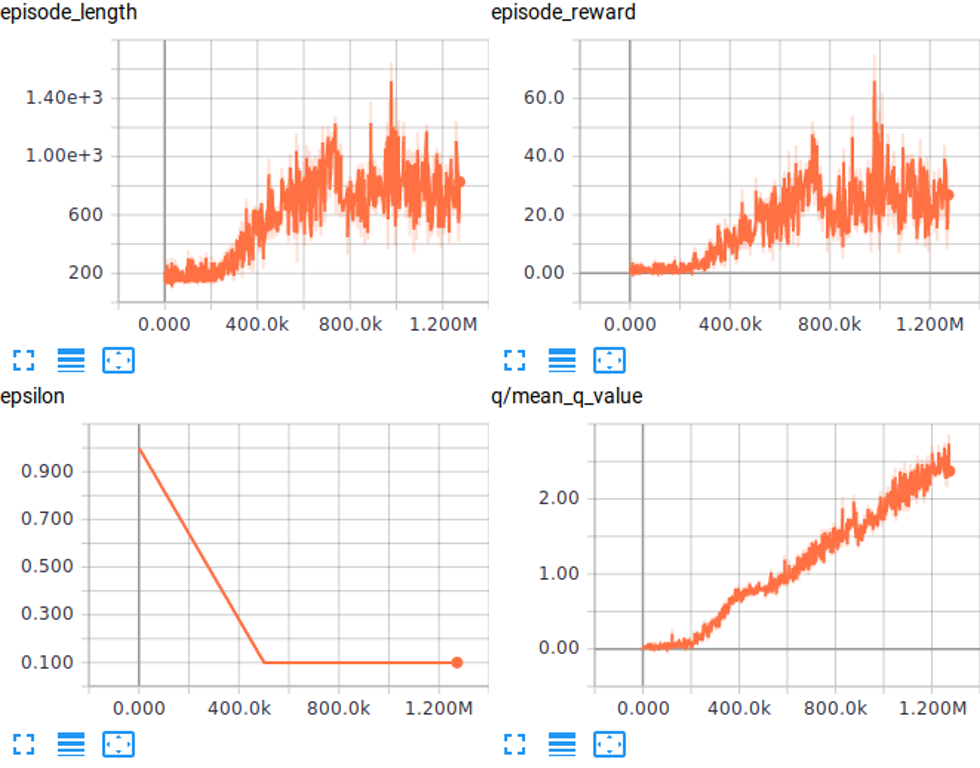Example of TensorBoard visualizations showing progress on training (on x axis is number of frames).

### Results

After about 3000 episodes of training, my agent is able to play the game quite well :)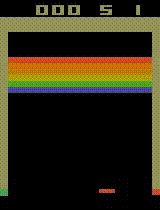Independent AI agent playing BreakOut after 3000 episodes of training

## Conclusion

In this post, I have demonstrated that reinforcement learning problems can be solved with reasonably simple, yet very powerful algorithms, I also pin-pointed some critical aspects of training a DL/RL model. The reinforcement learning field has made great leaps forward in past years and it appears to be staying on this track. I am personally really excited about applying RL in robotics and how will AI developed once it receives a physical body with means to experience and explore its environment.

Thanks for reading, everyone!

## Supplementary Info

### Takeaways

Below, I am listing some important personal takeaways. You will benefit from adopting them, especially if you didn’t do much of a practical DL/RL previously.

• Make sure you can fit a tiny dataset/simple problem
• Before adopting and adapting reference implementation, test that it works
• Make changes incrementally and test that they produce expected results. First get something that works, only then make it nice.
• For RL, you don’t need neither BatchNormalization nor Dropout. Regularization is in general much less important than in other settings.
• Visualize loss, metrics, parameter updates, Q-values, actions and more throughout the training (Callbacks and Tensorboard are your friends)
• Periodically visualize the agent as it plays
• Get proper hardware for training. Either good desktop or remote resources.

I also created a Reveal.js presentation where I go through these and other highly relevant and mainly practical aspects of deep learning models and training. Check it out here.